# PostgreSQL Mathematical Operators

## Mathematical Operators

PostgreSQL provided many Mathematical operators for common mathematical conventions. The bitwise operators can be used only with the integer data types.

List of Mathematical Operators

Operators Description Example Output
+ Addition 5 + 8 13
- Subtraction 6 - 9 -3
* Multiplication 5 * 8 40
/ Division 15 / 3 5
% Modulo ( Remainder ) 15 % 2 1
^ Exponentiation 4.0 ^ 2.0 16
| / Square Root | / 16 4
| | / Cube Root | | / 27 3
! Factorial ! 6 720
! ! Factorial ( with prefix operator) ! ! 6 720
@ Absolute Value @ -15.07 15.07
& Bitwise AND 53 & 38 36
| Bitwise OR 53 | 38 55
# Bitwise XOR 53 # 38 19
~ Bitwise NOT ~ 38 -39
<< Bitwise shift left 25 << 2 100
>> Bitwise shift right 120 >> 3 15

PostgreSQL Plus ( + ) operator example

If we want to add two numbers, the following SQL can be used.

SQL

Code:

``````SELECT 5+8 AS "Addition";
```
```

Output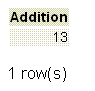PostgreSQL Minus ( - ) operator example

If we want to subtract two numbers, the following SQL can be used.

SQL

Code:

``````SELECT 6-9 AS "Subtraction";
```
```

Output: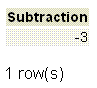PostgreSQL Multiplication ( * ) operator example

If we want to multiply two numbers, the following SQL can be used.

SQL

Code:

``````SELECT 5 * 8 AS "Multiplication";
```
```

Output: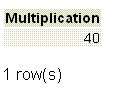PostgreSQL Division ( / ) operator example

If we want to division two numbers, the following SQL can be used.

SQL

Code:

``````SELECT 15 / 3 AS "Division";
```
```

Output: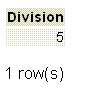PostgreSQL Modulo ( % ) operator example

If we want to get the remainder of a division by two numbers, the following SQL can be used.

SQL

Code:

``````SELECT 15 % 2 AS "Remainder";
```
```

Output:PostgreSQL Exponentiation ( ^ ) operator example

If we want to get the exponentiation of a number powered by another number, the following SQL can be used.

SQL

Code:

``````SELECT 4.0 ^ 2.0 AS "Exponentiation";
```
```

Output: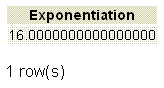PostgreSQL Square Root ( |/ ) operator example

If we want to get the square root of a number, the following SQL can be used.

SQL

Code:

``````SELECT |/16 AS "Square Root";
```
```

Output: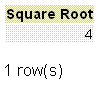PostgreSQL Cube Root ( | | / ) operator example

If we want to get the cube root of a number, the following SQL can be used.

SQL

Code:

``````SELECT ||/27 AS "Cube Root";
```
```

Output: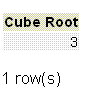PostgreSQL Factorial (!) operator example

If we want to get the factorial of a number, the following SQL can be used.

SQL

Code:

``````SELECT 6! AS "Factorial";
```
```

Output: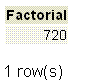PostgreSQL Factorial(with prefix) (! !) operator example

If we want to get the factorial of a number with factorial prefix operator, the following SQL can be used.

SQL

Code:

``````SELECT !!6 AS "Factorial ( with prefix )";
```
```

Output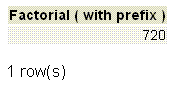PostgreSQL Absolute Value ( @ ) operator example

If we want to get the absolute value of a number, the following SQL can be used.

SQL

Code:

``````SELECT @ -15.07 AS "Absolute Value";
```
```

Output: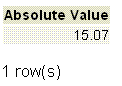PostgreSQL Bitwise AND ( & ) operator example

The PostgreSQL Bitwise AND ( & ) operator compares corresponding bits for each operand and produces a 1 when both bits are 1 otherwise it returns 0.

SQL

Code:

``````SELECT 53 & 38 AS "Bitwise AND";
```
```

Output: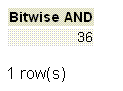Pictorial representation of PostgreSQL Bitwise AND ( & ) operator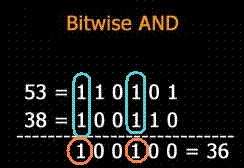PostgreSQL Bitwise OR ( | ) operator example

The PostgreSQL Bitwise OR ( | ) operator compares corresponding bits for each operand and produces a 1 when either or both bits are 1 otherwise it returns 0.

SQL

Code:

``````SELECT 53 | 38 AS "Bitwise OR";
```
```

Output: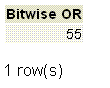Pictorial representation of PostgreSQL Bitwise OR ( | ) operator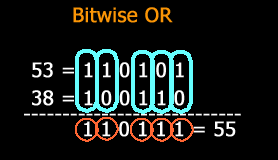PostgreSQL Bitwise XOR ( # ) operator example

The PostgreSQL Bitwise XOR ( # ) operator is similar to OR operator. It compares corresponding bits for each operand and produces a 1 when either but not both bits is 1 otherwise it returns 0.

SQL

Code:

``````SELECT 53 # 38 AS "Bitwise XOR";
```
```

Output: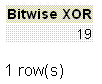Pictorial representation of PostgreSQL Bitwise XOR ( # ) operator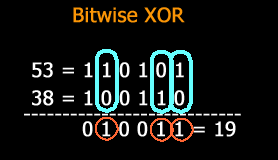PostgreSQL Bitwise NOT ( ~ ) operator example

The PostgreSQL Bitwise NOT ( ~ ) operator is a unary operator. It produces a toggled result, that is all 0's converted to 1 and all 1's are converted to 0.

SQL

Code:

``````SELECT ~38 AS "Bitwise NOT";
```
```

Output: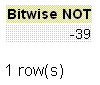PostgreSQL Bitwise Left Shift ( << ) operator example

The left shift(<<) operator shifts the bits in the first operand to the left by n bits, where n is the second operand. The left shift by n bits is similar to multiply the first operand by 2 to the power n.

SQL

Code:

``````SELECT 25 << 2 AS "Bitwise Left Shift";
```
```

Output: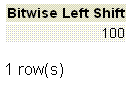Pictorial representation of PostgreSQL Bitwise left shift ( << ) operator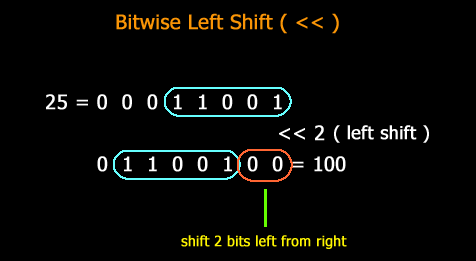PostgreSQL Bitwise Right Shift (>>) operator example

The right shift (>>)operator shifts the bits in the first operand to the right by n bits, where n is the second operand. A right shift by n bits is similar to dividing the first operand by 2 to the power n.

SQL

Code:

``````SELECT 120 >> 3  AS "Bitwise Right Shift";
```
```

Output: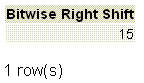Pictorial representation of PostgreSQL Bitwise right shift ( >> ) operator﻿